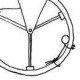Quora is one of my favorite time-waster websites, and somehow, I signed myself up to get a semi-weekly email from them that always seems to draw me into reading all the way down the email. Yesterday, I came across this question:Since we’ve been studying energy in my send year Matter and Interactions course, and just finished studying centripetal forces in the previous chapter, this seemed like a wonderful problem for our last class of 2017.

We quickly went to estimating the things we knew to solve this. We’ve talked about the solar constant before and we knew that at the radius of the earth, every square meter receives 1400W of light energy on average. Knowing that it takes light 8 minutes to reach the Earth from the Sun, we quickly calculated the total power of the earth to be around 10^26W, and so we knew that the sun was losing 10^26 J of mass energy every second.

We knew that this energy was coming from fusion and that the sun was losing rest energy. We found the energy using the idea$\Delta E =\Delta m c^2$$\Delta m = 1.1 \times 10^9 kg$

This was a pretty huge mass, but we weren’t as worried when we realized the mass of the sun is ~10^31 kg.

We then wondered how long the sun had been around, and figured a good estimate would be slightly longer than the earth, or about 4 billion years. Since we only care about the how the orbit of the earth might have changed, we wondered how much the mass of the sun changed while the earth was around. Googling 4 billion years in seconds gave us 10^17s, so the sun has lost about 10^26 kg of mass or around 100 Earths of mass.

Though this turns out to be only about 0.01% of the Sun’s mass, we still wondered if this would have a noticeable effect on the Earth’s orbit, and it was at this point that we realized computational modeling could give us an answer. We’ve already written a model of the earth-sun which includes code like this:

dt = 86400 #make time step 1 day while t < 4e9 * dt: #update forces Fg = (-Gm_sunm=_earth/r**2)*norm(r) #update momentum earth.p = earth.p+Fg*dt #update position earth.pos = earth.pos + earth.p/earth.m*dt 

And we realized that we could add a single line about the gravitational force calculation that read: m_sun = m_sun -1.1e9 *dt 

to account for the changing mass of the sun. We then thought we could write a program that plotted two earths—one feeling a force from a constant mass sun, and one feeling a force from a changing mass sun, and see how they departed.

All of this was great until we realized we’d set a timestep of a single day, and a wile loop that would need to run for 4billion years, and around this time, the class was almost over, but we thought of a few problems:

• We can’t increase the size of the time step by much because our approximation that the final momentum is equal to the average momentum is conditioned upon the idea that the net force is constant over the time interval
• Our errors from the time steps accumulate as time goes forward in in our program, so we weren’t even sure that a 1-day long time step would work for a 4 billion year long calculation

The beauty of this was we saw that this would be a nearly impossible problem to solve in closed form—integrating a force that depends on both position and changing mass seems daunting to me, but it’s totally do-able (in theory) computationally. We also all agreed that the answer is likely to be there is no discernable change in the orbit.

I’m sure there is some algorithm or method that would allow you to use a larger time step, or somehow quickly compute these trajectories, but I must admit I don’t know what it is off the top of my head, and would welcome ideas from my readers.

1.December 16, 2017 5:40 pm

Cool problem! I think you’ve done a good job explaining how to go about it and the problems with the approach. This is a very subtle effect, I would think, so tough to suss out.

I’d look at a simpler model: perfect circular orbit. Look at the result after 4 billion years at the full mass and do a worst case of 4 billion years at the end mass. You can calculate how many more “years” have gone by for the former case to see the upper bound of the difference.

Now, if you’re wondering about the change of shape of the orbit, which definitely would happen even if you started circular, then I think it gets trickier. Again you can get an upper bound (I think) by assuming that the mass change happens all at once. At that point the circular orbit would instantly become elliptical and you can compute the eccentricity of that upper bound.

I’ll put some more thought into how to do the computation without needing billions of time steps and let you know if I come up with anything.

2.December 17, 2017 9:53 pm

Pretty cool, although I can’t help you with your query. How are you going from dx = dv*dt = dp/m * dt in terms of orbit direction? Do you have code that deals with direction and vectors?